FAQ

# 1 General.

## 1.1 How can I submit an effective request for help?

Before submitting a request, first see if you can solve your own problem.

1. Check to see if you are using the latest version of pomp: if not, check to see if the problem you have is solved by upgrading to the latest version.
2. Before submitting a request, examine the error messages and try to figure out what they are telling you by reading the help pages and/or the package tutorials.

If you still have a problem, then submit a request for help via the Issues page if possible or to Aaron King (kingaa at umich dot edu) if necessary. Include in your request—at a minimum—the code that you ran that produced the error, together with a transcript of the R session that gave the errors. Better still, construct a minimal example that will reproduce the error: this allows for the most efficient solution of problems.

Make sure to include the output of following, so that I can see what version of pomp you are using, what version of R, what kind of machine you have, any customizations you have that might be affecting performance, etc.

source("https://kingaa.github.io/scripts/diagnostics.R")

## 1.2 What’s the proper way to cite pomp?

To cite the ‘pomp’ package in publications, use:

A. A. King, D. Nguyen, E. L. Ionides (2016). Statistical Inference for Partially Observed Markov Processes via the R Package pomp. Journal of Statistical Software 69(12): 1–43. DOI:10.18637/jss.v069.i12.

Additionally, consider citing the package itself:

A. A. King, E. L. Ionides, C. M. Breto, S. P. Ellner, M. J. Ferrari, S. Funk, S. G. Johnson, B. E. Kendall, M. Lavine, D. Nguyen, E. B. O’Dea, D. C. Reuman, H. Wearing, and S. N. Wood (2021). pomp: Statistical Inference for Partially Observed Markov Processes. (R package, version 3.5.2.0). https://kingaa.github.io/pomp/

You can get this information by executing citation("pomp") in an R session. LaTeX users can get a BibTeX entry for these publications by executing toBibtex(citation("pomp")).

If you use pomp in a publication, please take a moment to let us know! Send an email with the citation, and we’ll include it in the bibliography.

## 1.3 I have an error message that I don’t understand, and my question isn’t answered below. How can I get help?

The pomp developers have a goal of replacing all unhelpful error messages with helpful ones. Please help us while we help you! You can do this by submitting a help request via the package issues page. Display the error message you get and document how it arises. In doing so, please follow the instructions in FAQ 1.1 above.

## 1.4 I am running a piece of pomp code I found on the web, but I get errors. What is going on?

Of course, there could be many reasons for this. However, you should know that pomp version 2 made many changes to the pomp interface so that older code will not run under versions ≥ 2. See the pomp version 2 upgrade guide for information on how to fix broken code from earlier versions.

# 2 Installation problems

## 2.1 How do I install pomp?

See the installation instructions.

## 2.2 Why do I get an error message about gfortran when I try to install pomp from source on my Mac?

Compilation of pomp requires a FORTRAN compiler, which has not been installed (or not installed properly) on your system. See the installation instructions.

## 2.3 I am using R version X. When I try to install the package, I get the error that “package pomp is not available (for R version X).” Yet it is stated that pomp is compatible with R(≥Y), where X > Y. What is going wrong?

Firstly, as a general rule, it’s very probably a good idea to simply install the latest version of R, since each new release fixes bugs, adds and improves features, etc.

Usually, this warning arises because you are trying to install binaries from a repository on which binaries built for your version of R are no longer available. The preferred solution in this case is, again, to upgrade R, but if for some reason that is impossible or undesirable, you can always build the package from source using the source code retrieved from the package website or from one of the CRAN mirrors. For more, see the installation instructions.

To be clear: the statement that pomp will work for R(>=Y) is a guarantee that the code is compatible with those versions of R. It is not a promise that binaries will always be available for old versions of R.

# 3 Implementing POMP models.

## 3.1 How can I include a vector of variables in a C snippet?

A C snippet can make use of any feature of the C language. In particular, we can use pointers to give access to arrays. For example, consider the following, which implement a simple chain of random variables.

rSim <- Csnippet("
double *x = &X1;
int i, n = (int) N;
for (i = 0; i < n-1; i++) x[i] = x[i+1];
x[n-1] = rnorm(0,1);
")

rInit <- Csnippet("
double *x = &X1;
int i, n = (int) N;
for (i=0; i < n; i++) x[i] = 0.0;
")

rMeas <- Csnippet("
Y1 = rnorm(X2,2);
")

The following simulates and plots using the above.

library(ggplot2)
library(tidyr)

simulate(
times=1:20,t0=0,
rprocess=euler(rSim,delta.t=1),
rmeasure=rMeas,
rinit=rInit,
obsnames="Y1",
statenames=sprintf("X%d",1:5),
paramnames="N",
params=c(N=5),
format="data.frame"
) %>%
melt(id=c("time",".id")) %>%
ggplot(aes(x=time,y=value,group=variable,color=variable))+
geom_line()+theme_bw()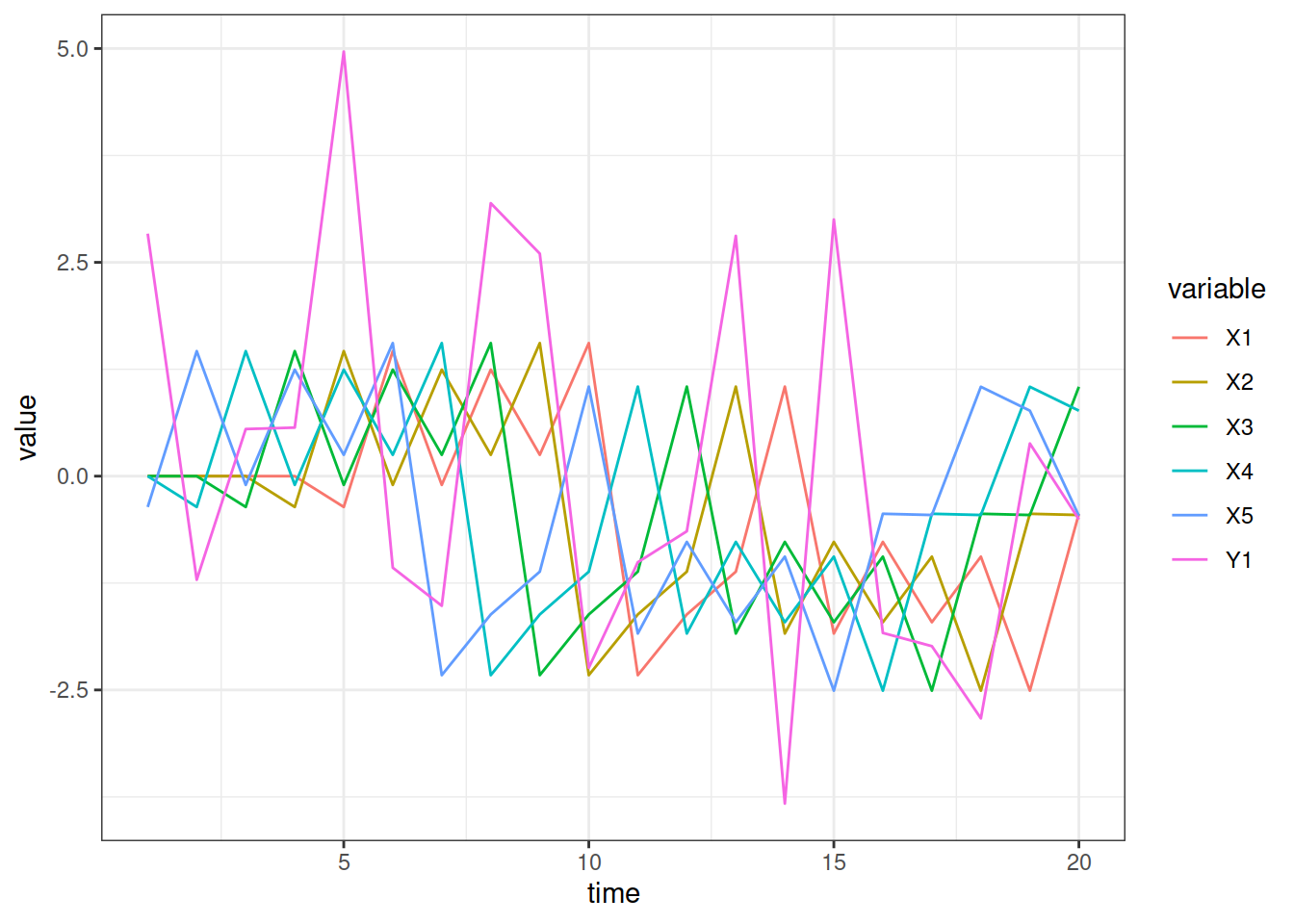## 3.2 Can I write a pomp for multivariate data?

Yes, this is no problem. The data you supply to pomp can contain multiple variables. You simply refer to these variables by name in writing the rmeasure and dmeasure C snippets. The following example illustrates this for a multivariate Ornstein-Uhlenbeck process (as in pompExample("ou2")) with a slightly peculiar measurement model.

simulate(
times=1:100, t0=0,
rmeasure=Csnippet("
y1 = rnorm(x1+x2,sigma1);
y2 = rnorm(x2-x1,sigma2);
"),
dmeasure=Csnippet("
lik = dnorm(y1,x1+x2,sigma1,1)+dnorm(y2,x2-x1,sigma2,1);
lik = (give_log) ? lik : exp(lik);
"),
rprocess=discrete_time(
step.fun=Csnippet("
double tx1, tx2;
tx1 = rnorm(a11*x1 + a12*x2,nu1);
tx2 = rnorm(a21*x1 + a22*x2,nu2);
x1 = tx1; x2 = tx2;
"),
delta.t=1),
rinit=Csnippet("
x1 = 0;
x2 = 0;
"),
obsnames=c("y1","y2"),
statenames=c("x1","x2"),
paramnames=c("a11","a12","a21","a22","sigma1","sigma2","nu1","nu2"),
params=c(a11=0.5,a12=-0.1,a21=0.2,a22=-1,nu1=0.3,nu2=0.1,sigma1=0.1,sigma2=0.3)
) -> obj

## 3.3 How do I deal with missing data?

Missing data are a common complication. pomp can handle NAs in the data, but the measurement model probability density function, dmeasure, if it is needed, must be written so as to deal with NAs appropriately. For example, look at the following variant of the SIR model describing the influenza outbreak in a boarding school:

library(magrittr)
library(ggplot2)

B,day
NA,0
1,1
6,2
26,3
73,4
NA,5
293,6
258,7
236,8
191,9
124,10
69,11
26,12
11,13
4,14
") -> dat

dat %>%
ggplot(aes(x=day, y=B)) +
geom_line() + geom_point()
## Warning: Removed 1 row(s) containing missing values (geom_path).
## Warning: Removed 2 rows containing missing values (geom_point).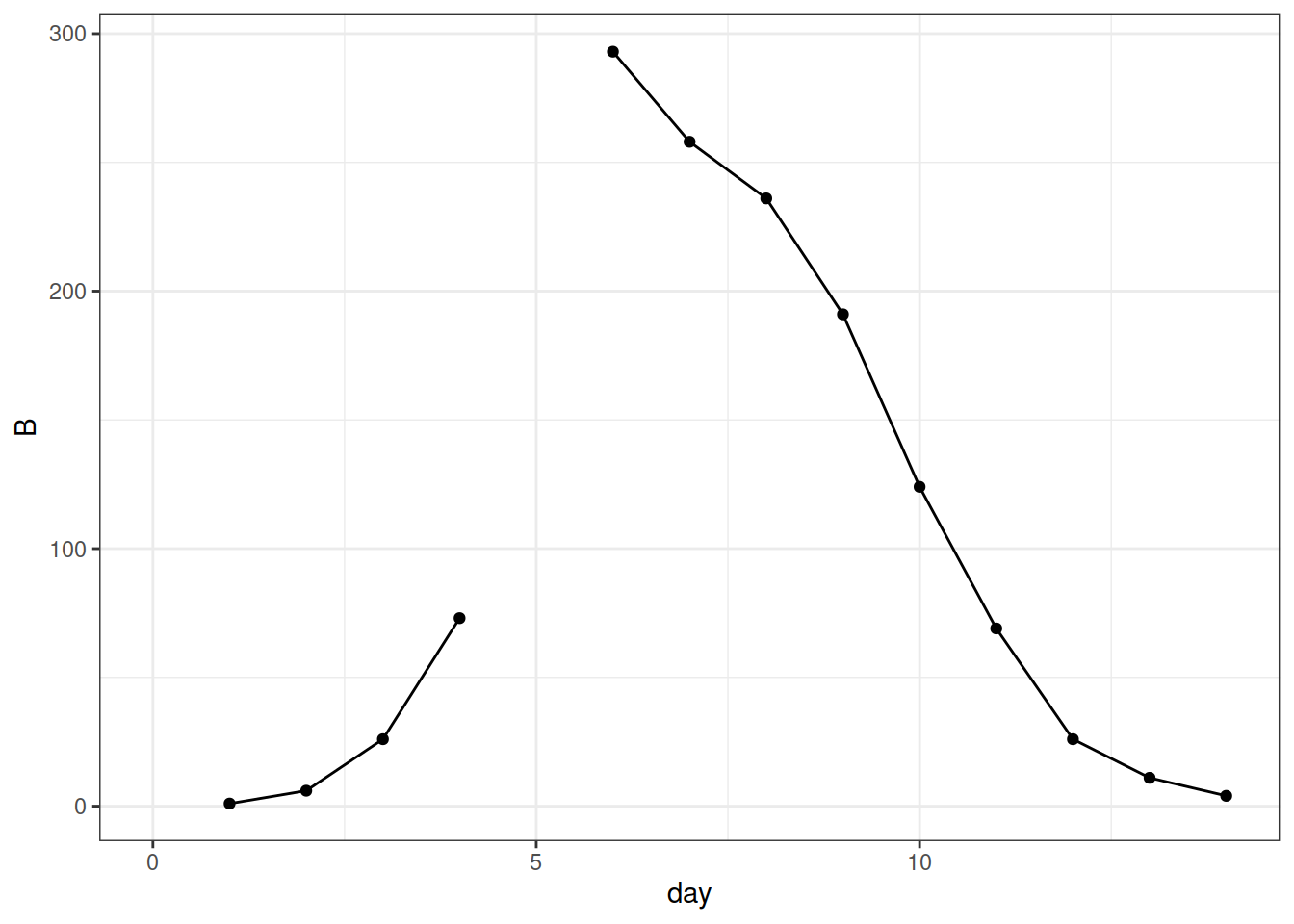Data are missing at days 0 and 5.

We create a pomp object implementing a simple SIR process model and a binomial measurement model, as in the original example. The only difference is in the dmeasure:

library(pomp)

sir_step <- Csnippet("
double dN_SI = rbinom(S,1-exp(-Beta*I/N*dt));
double dN_IR = rbinom(I,1-exp(-gamma*dt));
S -= dN_SI;
I += dN_SI - dN_IR;
R += dN_IR;
H += dN_IR;
")

sir_init <- Csnippet("
S = N-1;
I = 1;
R = 0;
H = 0;
")

rmeas <- Csnippet("B = rbinom(H,rho);")

dmeas <- Csnippet("
if (ISNA(B)) {
lik = (give_log) ? 0 : 1;
} else {
lik = dbinom(B,H,rho,give_log);
}
")

dat %>%
pomp(time="day",t0=0,
rprocess=euler(sir_step,delta.t=1/12),
rinit=sir_init,
rmeasure=rmeas,
dmeasure=dmeas,
accumvars="H",
paramnames=c("Beta","gamma","N", "rho"),
statenames=c("S","I","R","H")
) -> sir_na

In the above, to check for missing data, we use the ISNA macro, which is part of R’s C API. Note that the dmeasure returns a likelihood of 1 (log likelihood 0) for the missing data. [What’s the probability of seeing something if you don’t look?] When there is an observation, it returns a binomial likelihood as usual.

Our simulations now fill in simulated values for the missing data,

sir_na %>%
simulate(
params=c(Beta=3,gamma=2,rho=0.9,N=2600),
nsim=10,
format="data.frame",
include.data=TRUE
) %>%
ggplot(aes(x=day,y=B,group=.id,color=.id=="data"))+
geom_line()+
guides(color="none")+
theme_bw()
## Warning: Removed 1 row(s) containing missing values (geom_path).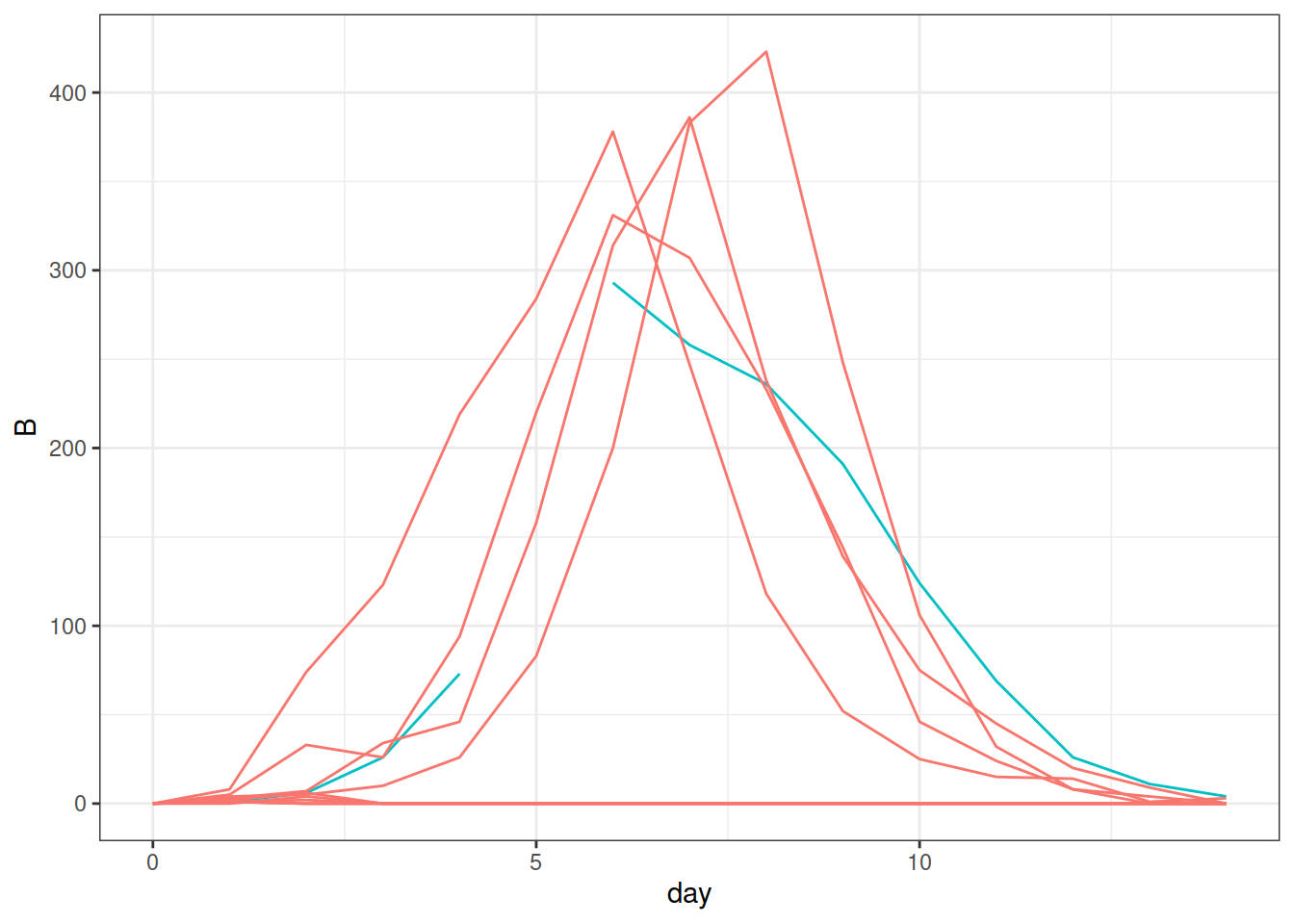and the particle filter handles the missing data correctly:

sir_na %>%
pfilter(Np=1000,params=c(Beta=3,gamma=2,rho=0.9,N=2600)) %>%
as.data.frame() %>%
melt(id="day") %>%
ggplot(aes(x=day,y=value,color=variable))+
guides(color="none")+
geom_line()+
facet_wrap(~variable,ncol=1,scales='free_y')+
theme_bw()
## Warning: Removed 1 row(s) containing missing values (geom_path).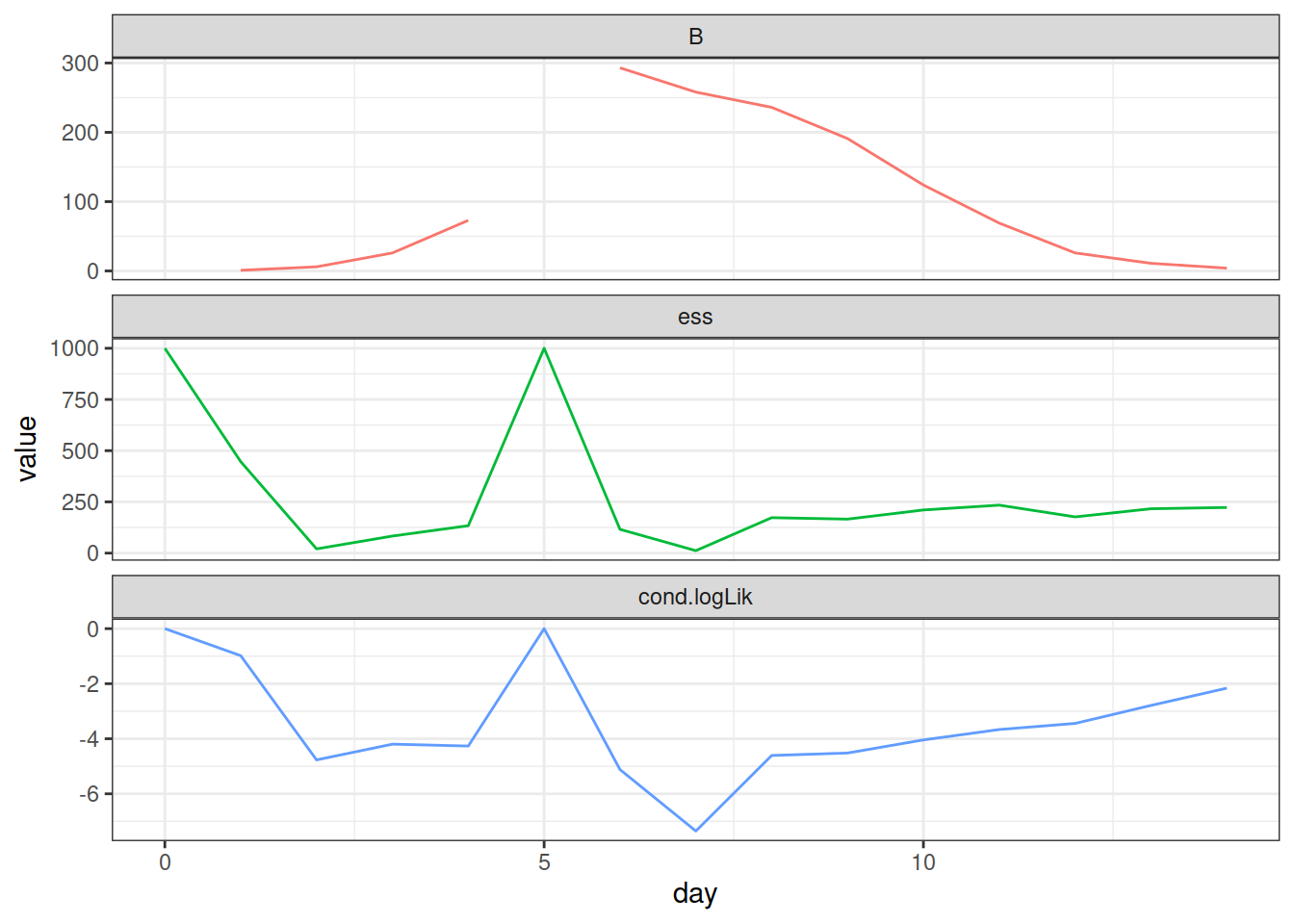In the above particle filter computation, notice that the effective sample size (ESS) is full, as it should be, when the missing data contribute no information.

## 3.4 I have t0 much less than t. How do I deal with accumulator variables in this case?

As described in the documentation (?pomp), pomp allows you to define “accumulator variables” that collect events occurring between observations. The example above illustrates this.

In that example, we took t0 equal to the first observation time. Sometimes we want to take t0 to be much earlier. For example, if we wish to posit that the initial state of the unobserved Markov process is distributed at t0 according to its stationary distribution, one way to achieve this is to put t0 so early that simulations will equilibrate before the first observation is made.

This is straightforward, but the presence of accumulator variables leads to a small twist. Let’s look at the boarding-school flu example to illustrate.

library(pomp)

sir_step <- Csnippet("
double dN_SI = rbinom(S,1-exp(-Beta*I/N*dt));
double dN_IR = rbinom(I,1-exp(-gamma*dt));
S -= dN_SI;
I += dN_SI - dN_IR;
R += dN_IR;
H += dN_IR;
")

sir_init <- Csnippet("
S = N-1;
I = 1;
R = 0;
H = 0;
")

rmeas <- Csnippet("B = rbinom(H,rho);")

dmeas <- Csnippet("if (ISNA(B)) {
lik = (give_log) ? 0 : 1;
} else {
lik = dbinom(B,H,rho,give_log);
}")

day,B
1,3
2,8
3,28
4,76
5,222
6,293
7,257
8,237
9,192
10,126
11,70
12,28
13,12
14,5
") -> dat

dat %>%
pomp(time="day",t0=-8,
rprocess=euler(sir_step,delta.t=1/12),
rinit=sir_init,
rmeasure=rmeas,
dmeasure=dmeas,
accumvars="H",
paramnames=c("Beta","gamma","N", "rho"),
statenames=c("S","I","R","H")
) -> bsflu

Note that, as above, we’ve allowed for the possibility of NAs in the data.

Now let’s look at the data and some simulations from the model.

library(ggplot2)

bsflu %>%
as.data.frame() %>%
ggplot(aes(x=day,y=B))+
geom_point()+geom_line()+
theme_bw()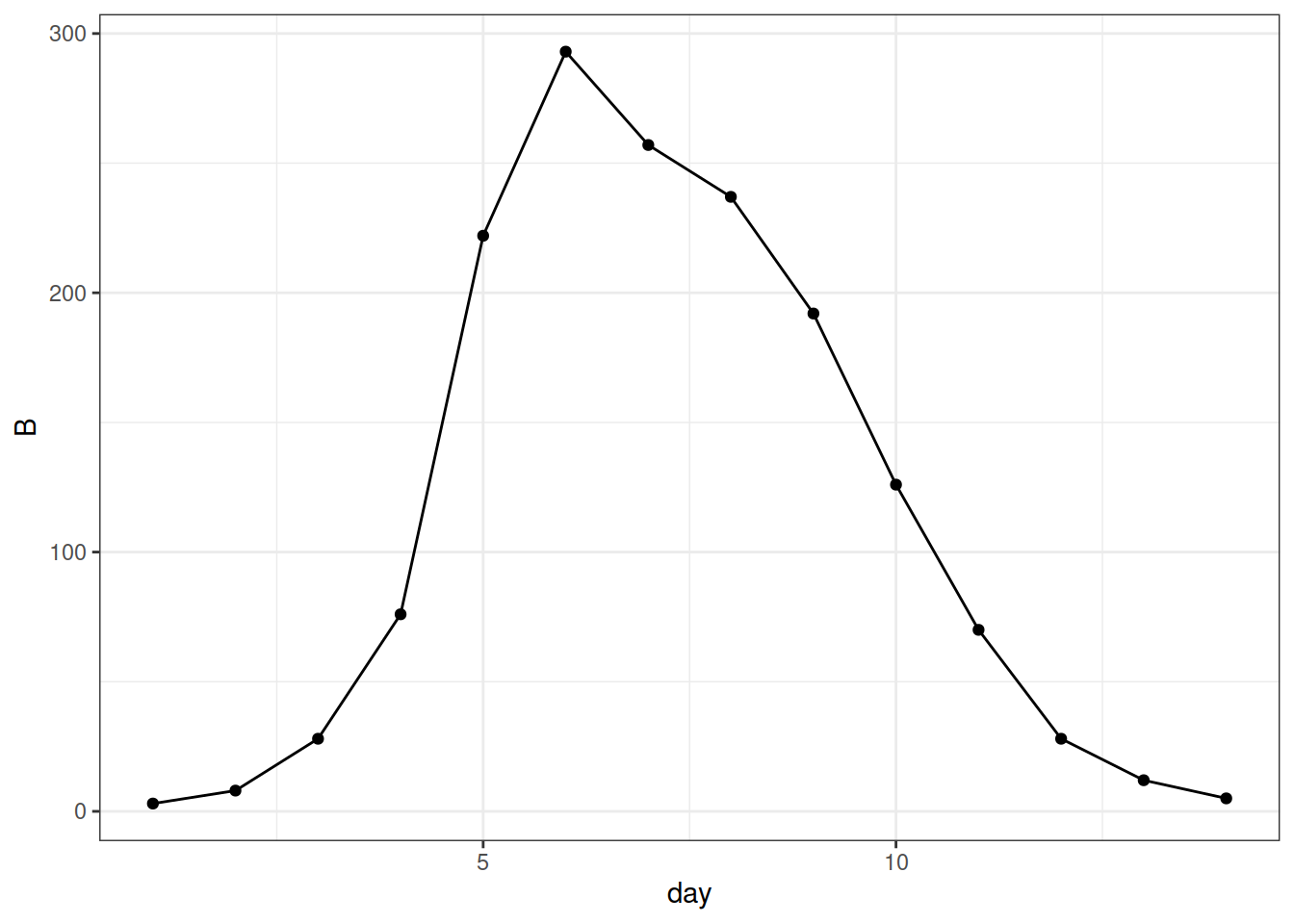bsflu %>%
simulate(params=c(Beta=1.5,gamma=1,rho=0.9,N=2600),
nsim=10,format="d",include.data=TRUE) %>%
ggplot(aes(x=day,y=B,group=.id,color=.id=="data"))+
geom_line()+
guides(color="none")+
theme_bw()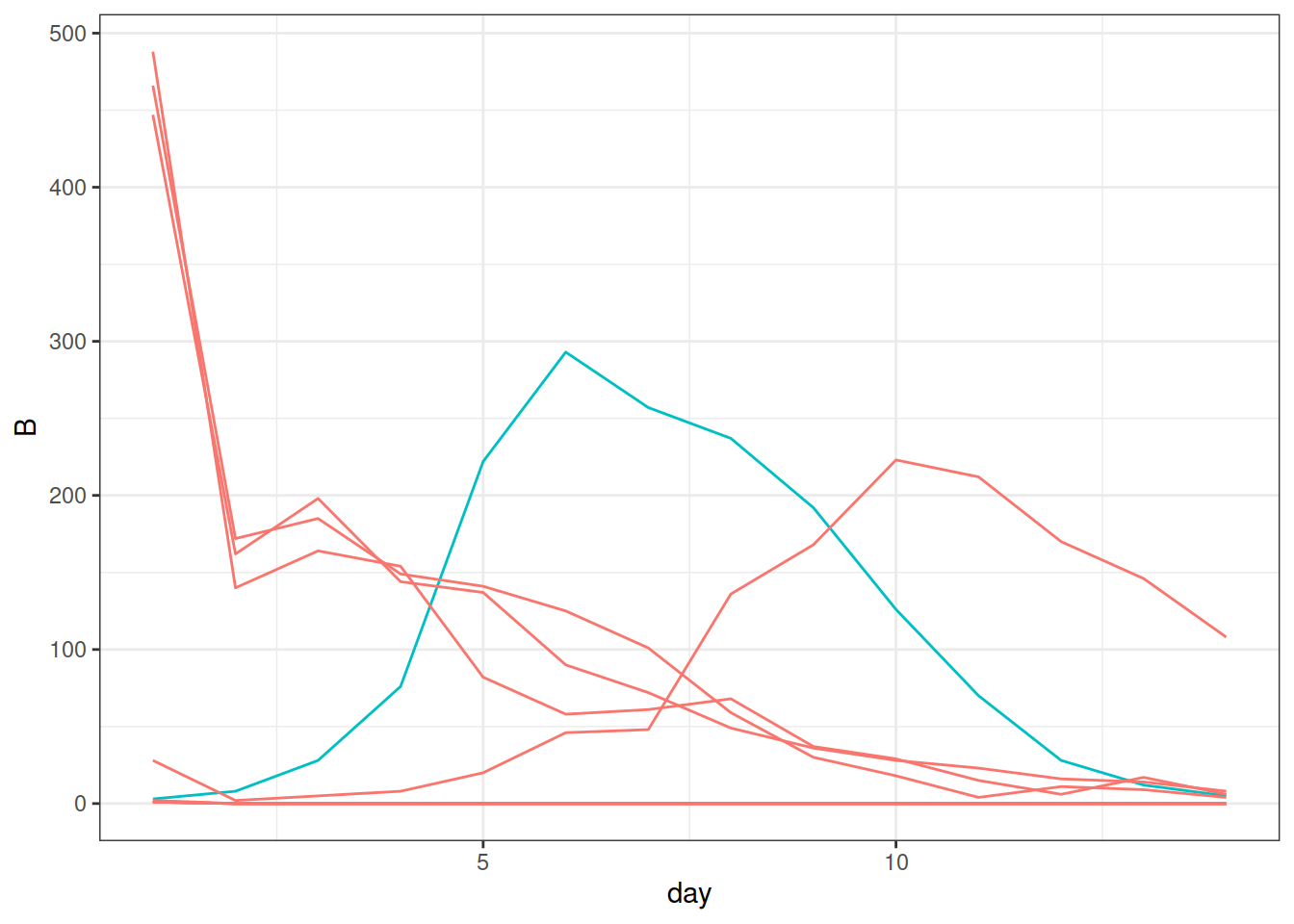The data plot looks fine, but what’s going on with the simulations? It’s easy to understand: we are assuming that there is one infectious host at t = t0 = -8 days. In most of the simulations, this leads to a number of new infections, which the H variable accumulates from t=-8 to t=1, the time of our first observation. But our first observation is not the number of new cases to have occurred in the last 9 days, but the number that occurred (and were reported) in the last 1 day.

We can fix this by introducing a fictitious “observation” at t=0, with missing data, i.e., by assuming we observed nothing at t=0:

library(dplyr)

dat %>%
bind_rows(c(day=0,B=NA)) %>%
arrange(day) %>%
pomp(time="day",t0=-8,
rprocess=euler(sir_step,delta.t=1/12),
rinit=sir_init,
rmeasure=rmeas,
dmeasure=dmeas,
accumvars="H",
paramnames=c("Beta","gamma","N", "rho"),
statenames=c("S","I","R","H")
) -> bsflu

bsflu %>%
simulate(params=c(Beta=1.5,gamma=1,rho=0.9,N=2600),
nsim=10,format="d",include.data=TRUE) %>%
ggplot(aes(x=day,y=B,group=.id,color=.id=="data"))+
geom_line()+
guides(color="none")+
theme_bw()
## Warning: Removed 1 row(s) containing missing values (geom_path).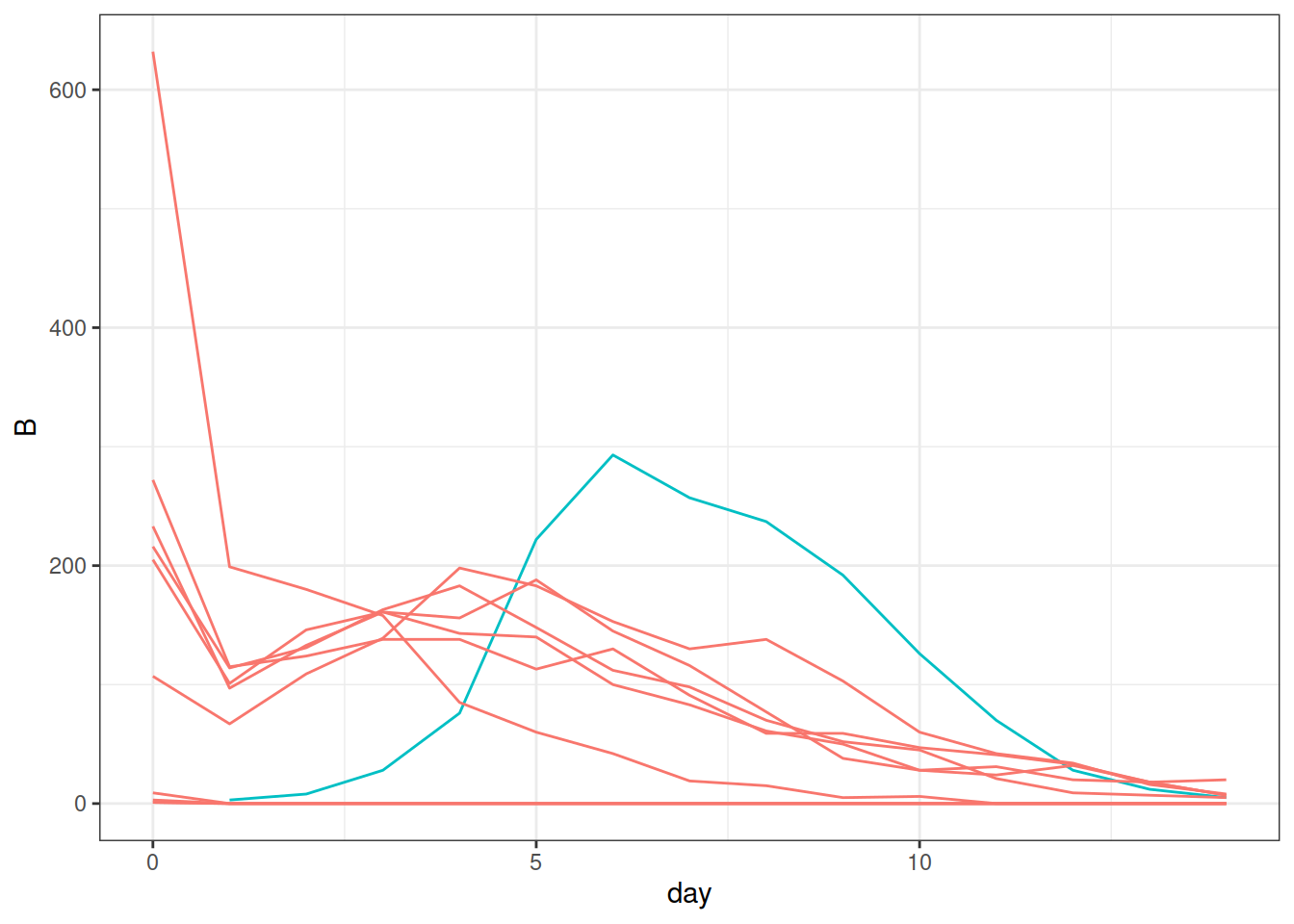## 3.5 What is the best measurement model to use?

The answer to this question depends on the nature of the data. The measurement model is as much a part of the model as the latent process model. Accordingly, the choice of the measurement model is governed by the same considerations: Which model fits the data best? Which model has the greater predictive power? Which model makes the most sense?

Nor is the choice of the measurement model without consequences for the choice of the latent process model. Despite the fact that one typically is much more interested in the latent process than in the measurement, if one does not pay careful attention to both, one risks making incorrect or sub-optimal inference.

## 3.6 What is the Euler-multinomial approximation?

The Euler multinomial approximation of a continuous-time, stochastic compartmental model is as follows. Suppose a compartment has occupancy $$N(t)$$ at time $$t$$ and that there are $$s$$ ways of exiting the compartment, with per capita rates (hazards) $$\mu_1,\dots,\mu_s$$, respectively.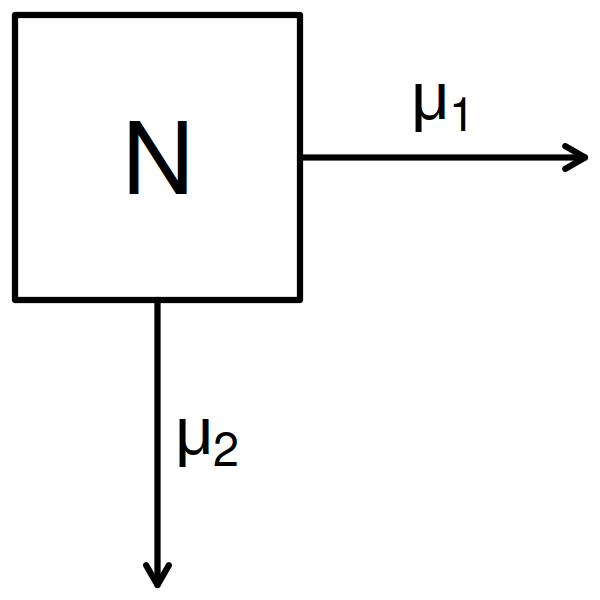Diagram: A single compartment within a compartmental model. Here, there are $$s=2$$ paths out of the compartment.

To make the Euler multinomial approximation, we approximate the total exit rates as constant over a small interval $$[t,t+{\Delta}t)$$. Let the random variable $${\Delta}n_k$$, $$k=1,\dots,s$$, be the number that exit by path $$k$$ in this time interval and $${\Delta}n_0$$ be the number that remain. Under this assumption, the vector of numbers of exits, $$({\Delta}n_{0},{\Delta}n_{1},\dots,{\Delta}n_{s})$$ is multinomially distributed with size $$N(t)$$ and probabilities $$(p_k)_{k=0}^s$$, where $p_0 = \exp\left(-\sum\!\mu_i\,{\Delta}t\right),$ and $p_k = \frac{\mu_k}{\sum\!\mu_i}\,\left(1-\exp\left(-\sum\!\mu_i\,{\Delta}t\right)\right),\qquad k=1,\dots,s.$ By way of shorthand, we say that $${\Delta}n=({\Delta}n_k)_{k=1}^s$$ is Euler-multinomially distributed with size $$N(t)$$, rates $$\mu=(\mu_k)_{k=1}^s$$, and time-step $${\Delta}t$$ and we write ${\Delta}n \sim \mathrm{Eulermultinom}\left(N(t),\mu,{\Delta}t\right).$

When constructing a POMP for a compartmental model using the Euler multinomial approximation, to write the rprocess basic component, one uses the euler plugin. One chooses the time step using the delta.t argument. In writing the step.fun C snippet, one needs exactly one call to reulermultinom for each compartment of the model. Using the notation above, one has to pack the $$s$$ rates $$\mu_1,\dots,\mu_s$$ into contiguous memory locations and retrieve the results in (a different set of) contiguous memory locations. For example, if rate is a pointer to $$s$$ contiguous memory locations holding the rates and dn is a pointer to $$s$$ contiguous memory locations ready to hold the results, then

reulermultinom(s,N,rate,dt,dn);

will result in a random sample from the Euler multinomial distribution (with timestep dt) being stored in dn, …, dn[s-1]. In the foregoing, we’ve assumed that the quantities $$N(t)$$ and $$s$$ are stored in the memory locations denoted N and s, respectively. Note that the rules for step.fun C snippets guarantee that the operative time step ($${\Delta}t$$) is stored in dt and that this will not exceed the user-specified delta.t. See ?rprocess_spec and ?Csnippet for more information.

When the step function is provided as an R function, the corresponding call is

dn <- reulermultinom(n=1,size=N,rate=mu,dt=delta.t)

where mu is a vector containing the rates.

# 4 Error messages and warnings.

## 4.1 I see an error about dmeasure returning illegal values. What does it mean?

A common error message is

Error : in ‘pfilter’: ‘dmeasure’ returns illegal value

This error arises because your dmeasure function is giving a negative, infinite, NA, or otherwise illegal value of the likelihood. Common causes are:

• negative or non-integer state variables when the dmeasure assumes non-negative or integer values,
• missing data,
• the dmeasure function or C snippet returning the log likelihood when the likelihood is requested, or vice versa.
• lik has not been set properly. Note that, when the snippet is executed, lik will be undefined. In particular, you cannot assume that it has value 0.

See the help (?Csnippet) for information on how to properly write dmeasure C snippets. As of version 1.5.6.3, when this error occurs, the values of the likelihood, parameters, and states are printed. If this alone doesn’t make the underlying cause clear, explore the model behavior further. A useful way to do this is to put Rprintf statements in your dmeasure and rprocess C snippets to view the values of the data, state variables, and likelihoods. Issue #13 has an example.

Remember, dmeasure has to be able to return the likelihood—or the log likelihood—upon request!

## 4.2 Why can’t I run the demos, or other codes that use the C snippet facility?

If your system does not support R CMD SHLIB, you won’t be able to use C snippets. R compiles native codes (C, C++, and FORTRAN) into shared-object libraries using SHLIB. To check whether your R installation is configured to use SHLIB, run the following code snippet in an R session:

cat(
"#include <R.h>
void hello (void) {
Rprintf(\"hello world!\\n\");
}",
file="hello.c")

system("R CMD SHLIB hello.c")

.C("hello",PACKAGE="hello")

If it works, you should see

hello world!
list()

If it doesn’t work, consult the installation instructions.

## 4.3 Why do I get an error message “Could not compile shared-object library” when I run pomp?

Also, some Windows users have reported trouble with certain parallel codes. Specifically, pomp codes that require compilation of C snippets trigger errors when executed in parallel on certain Windows machines.

The workaround is to execute serially all codes that require compilation; that is, to execute them outside of all parallel code blocks.

Calls that induce compilation include:

1. Every call to a pomp function in which a basic model component is specified using a C snippet. Recall that the basic model components include rmeasure, dmeasure, rprocess, dprocess, skeleton, rprior, dprior, partrans, and rinit. Whenever one of these arguments is furnished a Csnippet, the snippet is compiled.
2. Every call to a pomp function in which a parameter transformation is specified by giving the names of parameters to log-transform, logit-transform, or log-barycentric transform. Even though the user does not supply a Csnippet in such cases, each such call results in a Csnippet being written internally, and then compiled.

In addition, the use of temporary directories to hold the C files and dynamically loadable libraries created from them that underlie the C snippet facility causes problems on certain Windows machines. We hypothesize that this is due to Windows securities features.

Again, there is a workaround. One can specify to pomp that all compilation should be done in the current working directory.

An illustration is given in the Simulation-based Inference for Epidemiological Dynamics short course.

## 4.4 I get the error message ‘could not find symbol “recursive” in environment of the generic function’ when I use the c operator to concatenate certain kinds of pomp structures. How do I fix this?

This appears to arise from incompatibility between certain R installations and binary versions of the pomp package from CRAN. Try installing the latest version from the github site:

install.packages("pomp",repos="https://kingaa.github.io/")

If you continue to see the error, try installing from source. The easiest way to do this is to use devtools:

devtools::install_github("kingaa/pomp")

For more help with installation, see the installation instructions.

## 4.5 I get warnings about extrapolation in ‘table_lookup.’ What is this about?

pomp incorporates time-varying covariates via a lookup table. Whenever your model needs the value of a covariate at a particular time, it uses the lookup table provided (via the covar and tcovar options of pomp), linearly interpolating if necessary. It can happen that the required computation depends on values of the covariates outside the time interval covered by the lookup table. When this happens, pomp does linear extrapolation, with a warning.

This can be a problem if the linear extrapolation is not accurate. To avoid this, be sure to give values for the covariates over a time interval that covers the full range of relevant times, i.e., t0 to the time of the last observation.

Note that the ODE integration algorithms underlying trajectory can sometimes evaluate the covariates beyond the last observation time. To avoid warnings (and the potential inaccuracy they signal) in this case, it may be necessary to supply values for the covariates somewhat beyond the final observation time.

## 4.6 When trying to use C snippets, I get an error message that refers to files and directories that don’t exist. What is going on?

See Issue 115 for an example. This can happen if you use file or directory names that contain whitespace. For the tools that compile the C snippets—as with (all?) other command-line tools—whitespace is syntactic. By far the best solution to this problem is to avoid using whitespace altogether in file and directory names! The standard choice is to substitute the underscore "_" for whitespace.

## 4.7 ‘Warning: “beta” redefined.’

Error messages containing the following elements often arise.

...
Error: error in building shared-object library from C snippets:
in ‘Cbuilder’: compilation error: cannot compile shared-object library
...
compiler messages:
...
warning: "beta" redefined
...

This is due to a name conflict between a quantity beta defined as a model parameter, covariate, data variable, or in some other way defined in a C snippet, and the Euler beta function, which is defined as beta in Rmath.h and documented in the R extensions manual. To work around this, simply rename the parameter. For example, it could be called Beta instead.

## 4.8 I get an error saying X “must be a function of prototype” Y. What does this mean?

The error message displays the “prototype” of the function pomp expects. For example, in the message

Error : in 'sannbox': 'candidate.dist' must be a function of prototype 'candidate.dist(par, temp, scale, ...)'.

the prototype expected is candidate.dist(par, temp, scale, ...). This means that a function supplied to the candidate.dist argument must have at least the arguments par, temp, scale, and .... Note that the ellipses (...) are necessary. Similarly, the error message

Error: in ‘rmeasure’: ‘rmeasure’ must be a function of prototype ‘rmeasure(...)’

tells us that we have left the ... out of the argument list in the function we supplied for rmeasure.

# 5 Interpreting results

## 5.1 Is the likelihood mif2 gives me the same as the likelihood I get from pfilter?

No. As its name suggests, the iterated filtering (IF) algorithm (that implemented in pomp as mif2) work by iteratively performing particle filtering on a model, but that model is slightly different from the focal model. In particular, IF pertubs the parameters of the model by adding random noise to them. Actually, if one were to simulate from this model, the parameters would be taking random walks. This effectively smooths the likelihood surface, combats particle depletion, and—critically for IF—allows local exploration of the parameter space. The likelihood that mif2 reports is the estimated likelihood of this perturbed model. It can be either higher or lower than that of the focal model. Iterated filtering works by gradually reducing the magnitude of the perturbations with successive iterations (“cooling”), so that the perturbed model gradually becomes more similar to the focal model.

To compute the likelihood at a point returned by mif2, it is necessary to re-run the particle filter. By doing the latter several times, one can obtain a more accurate estimate of the likelihood along with a measure of the associated uncertainty.

## 5.2 Why does the likelihood seem to be decreasing, not increasing, with mif2 iterations?

In Issue 27, @theresasophia asked this question. She provided codes for an estimation procedure based on mif2. The plot below illustrates what she found.